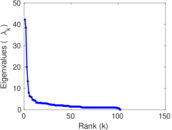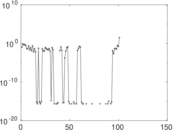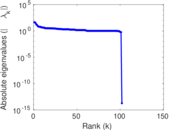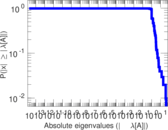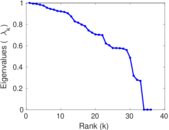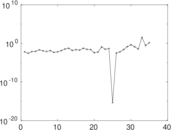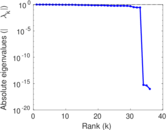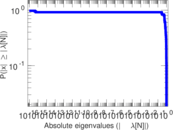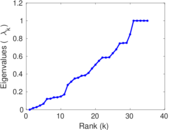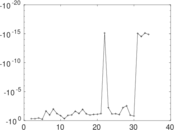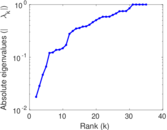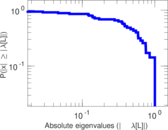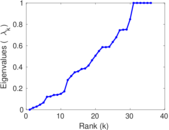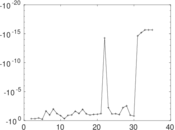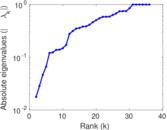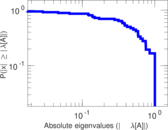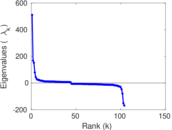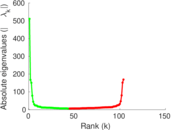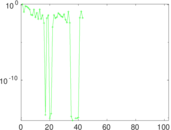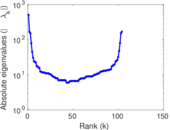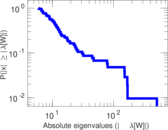# Wikibooks edits (zh-min-nan)

This is the bipartite edit network of the Chinese (Min Nan) Wikibooks. It contains users and pages from the Chinese (Min Nan) Wikibooks, connected by edit events. Each edge represents an edit. The dataset includes the timestamp of each edit.

 Code `bzh-min-nan` Internal name `edit-zh_min_nanwikibooks` Name Wikibooks edits (zh-min-nan) Data source http://dumps.wikimedia.org/ AvailabilityDataset is available for download Consistency checkDataset passed all tests Category Authorship network Dataset timestamp 2017-10-20 Node meaning User, article Edge meaning Edit Network formatBipartite, undirected Edge typeUnweighted, multiple edges Temporal dataEdges are annotated with timestamps

## Statistics

 Size n = 520 Left size n1 = 104 Right size n2 = 416 Volume m = 750 Unique edge count m̿ = 477 Wedge count s = 12,003 Claw count z = 480,911 Cross count x = 16,034,611 Square count q = 67 4-Tour count T4 = 49,774 Maximum degree dmax = 152 Maximum left degree d1max = 152 Maximum right degree d2max = 42 Average degree d = 2.884 62 Average left degree d1 = 7.211 54 Average right degree d2 = 1.802 88 Fill p = 0.011 025 3 Average edge multiplicity m̃ = 1.572 33 Size of LCC N = 315 Diameter δ = 14 50-Percentile effective diameter δ0.5 = 4.098 09 90-Percentile effective diameter δ0.9 = 7.969 70 Median distance δM = 5 Mean distance δm = 5.006 92 Gini coefficient G = 0.630 173 Balanced inequality ratio P = 0.252 000 Left balanced inequality ratio P1 = 0.196 000 Right balanced inequality ratio P2 = 0.352 000 Relative edge distribution entropy Her = 0.858 121 Power law exponent γ = 5.779 40 Tail power law exponent γt = 2.781 00 Tail power law exponent with p γ3 = 2.781 00 p-value p = 0.047 000 0 Left tail power law exponent with p γ3,1 = 1.891 00 Left p-value p1 = 0.724 000 Right tail power law exponent with p γ3,2 = 3.591 00 Right p-value p2 = 0.194 000 Degree assortativity ρ = −0.196 150 Degree assortativity p-value pρ = 1.598 08 × 10−5 Spectral norm α = 42.201 9 Algebraic connectivity a = 0.017 665 6 Spectral separation |λ1[A] / λ2[A]| = 1.098 70 Controllability C = 316 Relative controllability Cr = 0.610 039

## Plots

### Fruchterman–Reingold graph drawing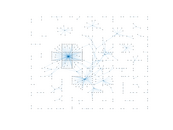### Degree distribution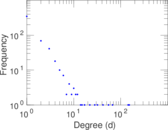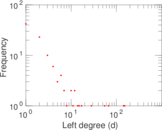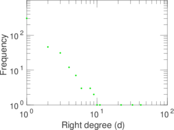### Cumulative degree distribution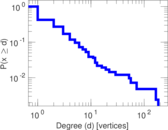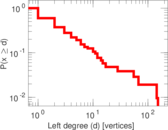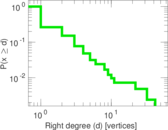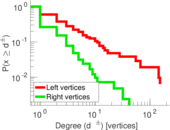### Lorenz curve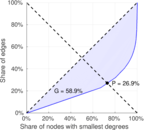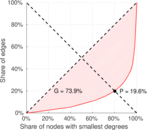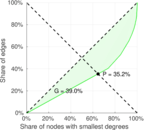### Spectral distribution of the adjacency matrix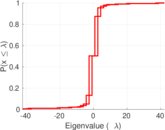### Spectral distribution of the normalized adjacency matrix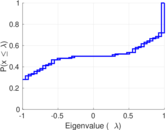### Spectral distribution of the Laplacian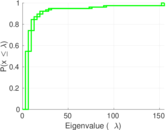### Spectral graph drawing based on the adjacency matrix### Spectral graph drawing based on the Laplacian### Spectral graph drawing based on the normalized adjacency matrix### Degree assortativity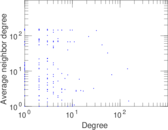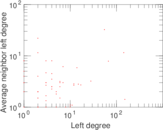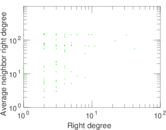### Zipf plot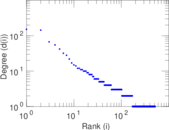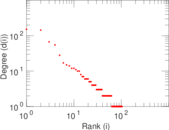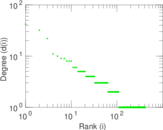### Hop distribution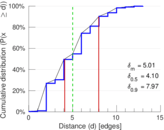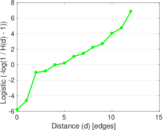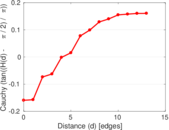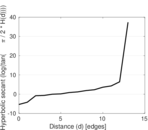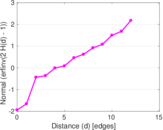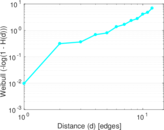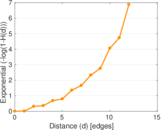### Double Laplacian graph drawing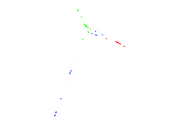### Delaunay graph drawing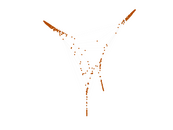### Edge weight/multiplicity distribution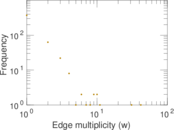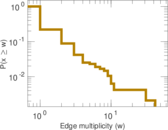### Temporal distribution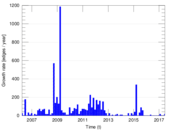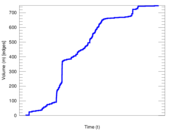### Temporal hop distribution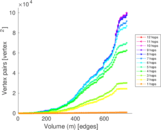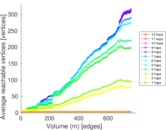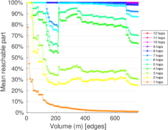### Diameter/density evolution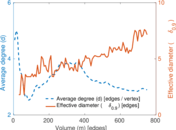### Matrix decompositions plots